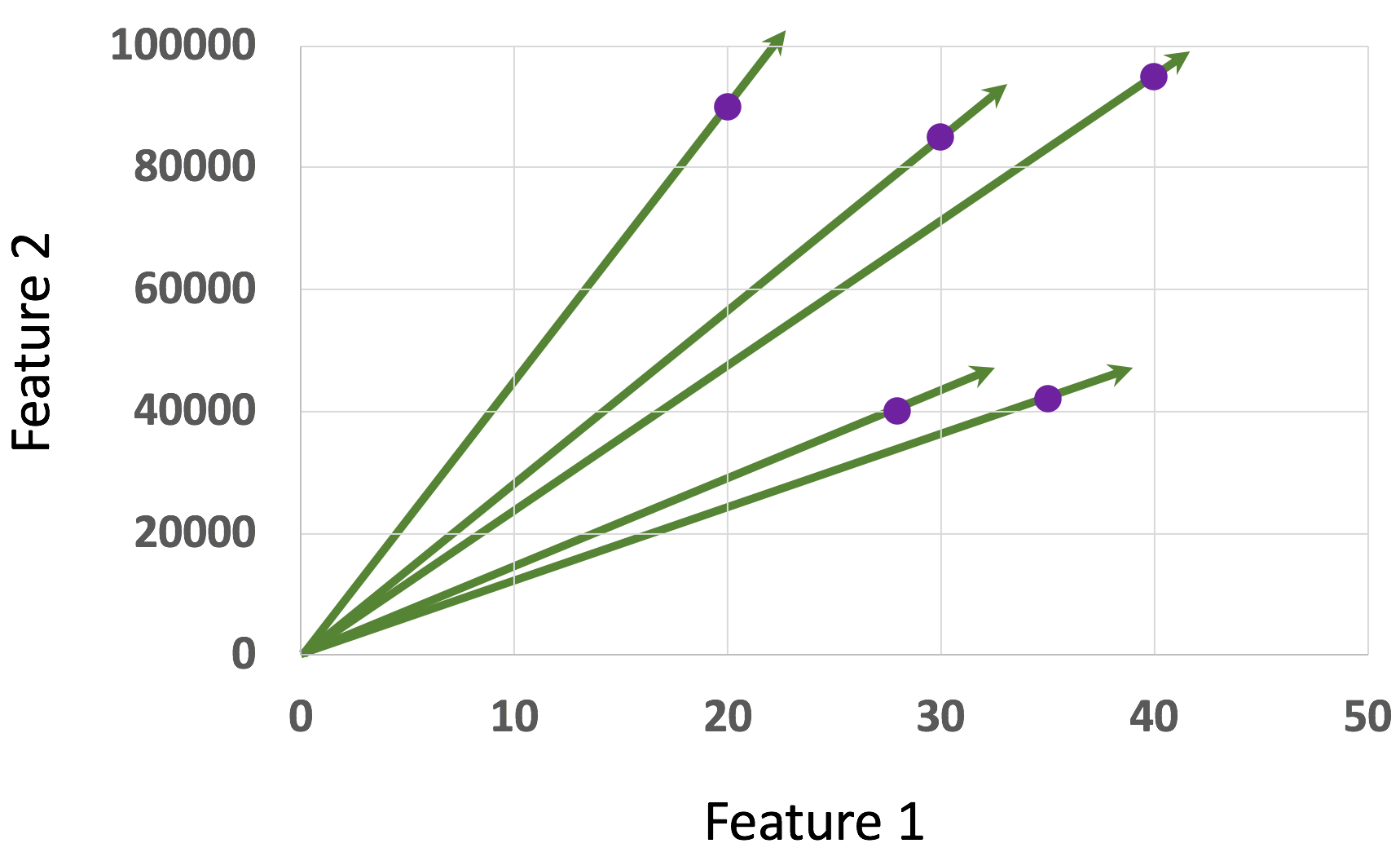• ##### Clustering algorithms
No items in this section

## Introductory Data Science

### What is a vector?

The word “vector” in physics refers to a quantity with a direction. A vector in data science is no different than a vector in physics. Each object or a data point of a tabular data is sometimes referred to as a vector.

That is, In data science,

a point in the space=an object of the data table=a vector

Why do we call an object a vector? Before answering this question, we need to know what an origin is.

## The origin

Regardless of the data points in the space, there is an origin in every data table. The origin is the coordinate where the value in every axis is zero. That is, the origin in a two-dimensional space is (0, 0). The origin in a three-dimensional space is (0, 0, 0). The origin of a four-dimensional space is (0, 0, 0, 0). So and so forth. There might not be an exact row in the table that contains all zeros, but there is one virtually in the space that is formed by the dataset.

## What is a vector?

A data point or an object is called a vector because it resembles the concept of direction and magnitude in physics or mathematics. The vector formed by a data point has the direction pointing from the origin toward the data point. The magnitude of the vector is considered to be the distance between the origin and the point.

Consider that we have the following two-dimensional data table.

 Feature 1 Feature 2 20 90000 30 85000 28 40000 40 95000 35 42000

We have five objects or five data points. Each of these five data points is a vector. All five vectors are drawn in the following figure. Notice that the vectors have a direction from the origin toward the data points.In practice, we do not have to worry much about the directions of the vectors in data science. Consider each vector as a one-dimensional array.

In computer science, an array is frequently called a vector because the array content forms a vector in the space. In data science, sometimes we use vector-based math, many times we don’t. For simplicity, you can consider that “vector” is another name of a data point or an object.

0 replies